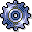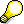Key Figures in Product Cost by OrderUse

The following cost objects are used in Product Cost by Order:

A large number of key figures are available in Product Cost by Order. You can display key figures:

In the report categories object lists and object comparisons

Which key figures can be analyzed depends on the object. For general information on the key figures in Cost Object Controlling, see the following:

Functions of Cost Analysis

Reports of cost analysis use the functionality of the ABAP List Viewer while offering additional functions in cost analysis. You can define layouts in cost analysis containing the key figures that you want to analyze. The column selection for the layouts contains different field groups (column lists) that separate the key figures into logical groups.

In Product Cost by Order, you can analyze:

• Target costs
• Control costs
• Individual variance categories
• Absolute and percentage variancesNote on Summarized Analysis with Summarization Hierarchy

If variances are calculated for an order (product cost collector or manufacturing order), the system sets the status VCAL (variances calculated) on the order. To assure comparability, you should only summarize objects for which variances have been calculated. Use the status selection profile in the required data collection. Select the orders for which the status VCAL is active.

If objects with the settlement type periodic or full are summarized together and analyzed by period, this should be taken into account when the report is interpreted.

See the following sections for information on product drilldown reports:

See the following sections for information on object lists:

Information on Object Comparisons: